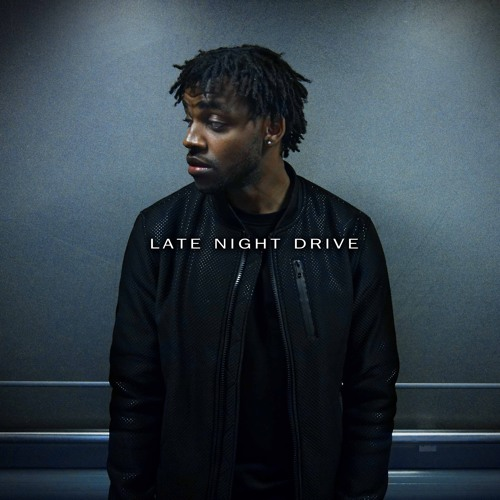# Yo Trane - Late Night Drive (EP)

Started by gavin, Nov 6, 2015, in Music

1. Nov 6, 2015=•=•=•=•=•=•=•=•=•=•=•=•=•=•=•=•=•=•=•=•=•=•=•=•=•=•=•=•=•=•=•=•=•=•=•=•=•=•=•=•=•=•=
Yo Trane is a 21-year-old French singer-songwriter which focuses on crafting a strong trap-influenced R&B sound, making his smooth vocals and ability to to sing high notes softly go straight to your soul.

Popular music is often bright and sunny, while this project is more nocturnal and emotional,” explains Yo Trane of the 7-track project which was entirely produced by Kalim. “Late Night Drive is aimed at the people who have trouble sleeping at night so they would rather hang out with their lovers or take a spin in their whips to remedy the insomnia.”
=•=•=•=•=•=•=•=•=•=•=•=•=•=•=•=•=•=•=•=•=•=•=•=•=•=•=•=•=•=•=•=•=•=•=•=•=•=•=•=•=•=•=
=•=•=•=•=•=•=•=•=•=•=•=•=•=•=•=•=•=•=•=•=•=•=•=•=•=•=•=•=•=•=•=•=•=•=•=•=•=•=•=•=•=•=
Now let's begin the adventure through this beautiful project.

Before anybody ask, no this is not me I am simply a fan and sharing a up and coming artist with the people.

Last edited: Nov 6, 2015
2. Nov 7, 2015
Moved to music since this is a more established artist and not a SXN80 artist.

3. Nov 7, 2015
In my opinion the Weeknd is the King of R&B music rn but this guy could be his distant cousin because this ain't bad at all. @Sign Language

2
gavin and Cyreides like this.
2
gavin and Cyreides like this.
4. In my city, I'm a young God

Nov 7, 2015
Can't listen to it right now.
Does he speak English?
Does he have a heavy accent?
Can he provide a car and license so I can take a spin in the whip to remedy the insomnia?

Last edited: Nov 7, 2015
1
Ordinary Joel likes this.
1
Ordinary Joel likes this.
5. Aug 22, 2017
Alone Out Now!!